Scrapbook - Zonohedra 16 images tree :: Geometry > Scrapbook - Zonohedra
In This Album:

 Image List Thumbnail Page
Zonohedra can be generated by taking a set of vectors (a star), calculating the displacements produced by all possible combinations of these vectors, and then calculating the convex hull of these displacements.

Polar zonohedra are produced from the star of the slanting edges of a right regular pyramid. Symmetric zonohedra can be produced by vectors taken from the edges and vertices of uniform polyhedra.

 thumbnails 1/1
 Icosahedron all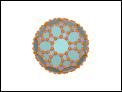Med  Lrg
 Icosahedron edges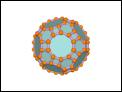Med  Lrg
 Icosahedron verticesMed  Lrg
 Polyhedron verticesMed  Lrg
 Polyhedron verticesMed  Lrg
 Cube verticesMed  Lrg
 Cuboctahedron vertices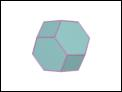Med  Lrg
 Icosahedron verticesMed  Lrg
 Polar Zonohedron 6Med  Lrg
 Polar Zonohedron 7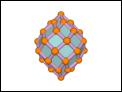Med  Lrg
 Polar Zonohedron 10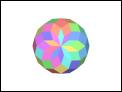Med  Lrg
 Polar Zonohedron 20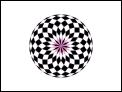Med  Lrg
 Polar Zonohedron 100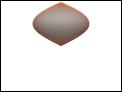Med  Lrg
 Polar Zonohedron 100Med  Lrg
 Polar Zonohedron 50Med  Lrg
 Polar Zonohedron 50Med  Lrg
 1